Select Page

I love Tabasco and always though that their cute little miniature bottles could be the basis for a great specialty lamp. I was very happy to find that a 3mm LED fits perfectly in the bottle opening of the miniature bottles. By designing a custom circuit board I was able to make a lamp that looks like a bundle of dried peppers.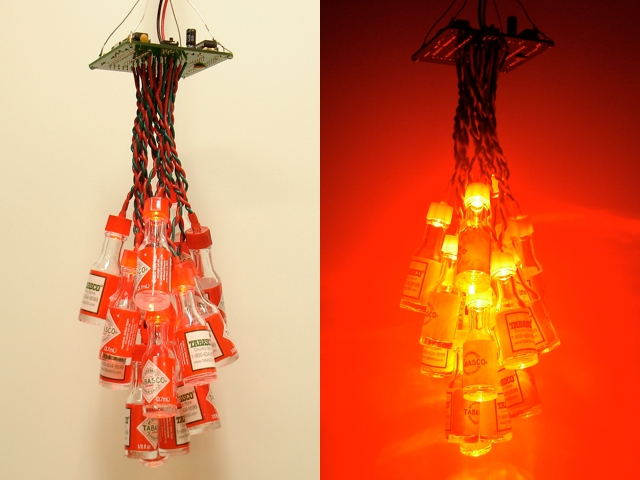Each bottle is individually controlled with a TLC5940 16-channel PWM driver. The software running on the ATmega328 is Arduino code that implements different lighting effects. Here it is in action:

### Hardware

I designed a circuit board for the ATmega328 and the TLC5940. This is basically a custom Arduino and the design files are available below in case you want to make one. The LEDs connect from the bottom of the board. I am using a 5V regulated power supply, so there’s no need for an on-board voltage regulator. I included a 6-pin serial header for easy Arduino programming and mounting holes on the corners so I can hang it from wires. I also included a tactile button in case I wanted to allow some input to control the lamp (currently unused). Also note the Tabasco logo right on the board silkscreen!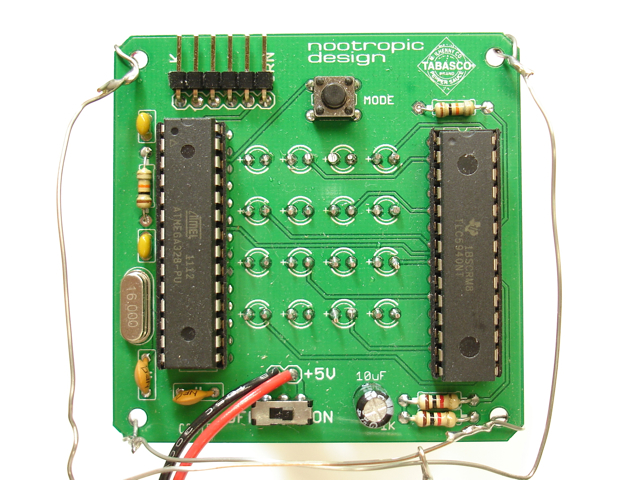I photographed the assembly process so you could see how I constructed the lamp.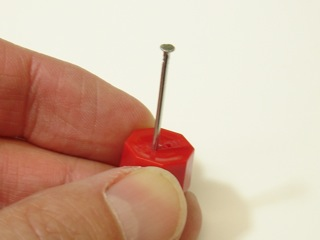1. Make two holes in each cap using a small nail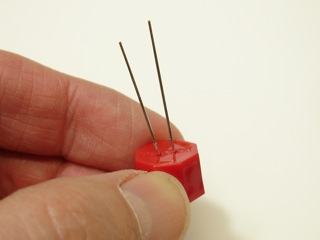2. Insert a 3mm LED into the cap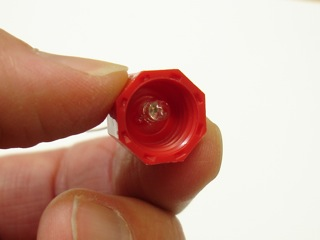3. Ensure the LED is in the center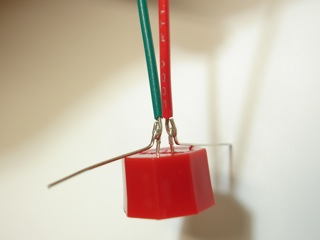4. Connect wires by bending the leads. Make sure they don’t short!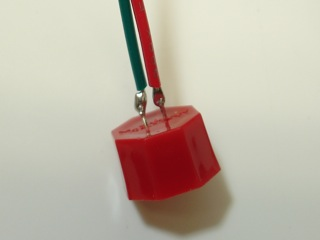5. Carefully solder and clip the leads.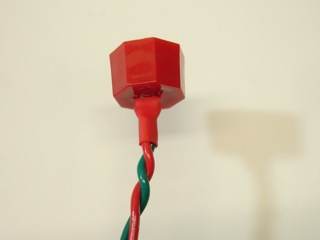6. Enclose in heat shrink tubing.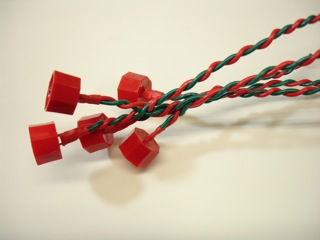7. Twist wires and weave together.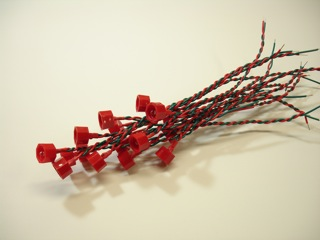8. Keep going!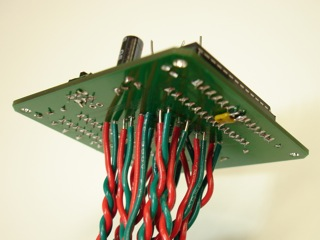9. Insert wires into underside of board.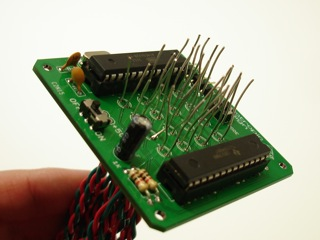10. Now just solder and clip the wires.

### Software

Here is the Arduino code running on the lamp. I used the TLC5940 Arduino library to control the PWM driver. You can download the code from here.

```#include "Tlc5940.h"

#define NPINS 16
#define NVISUALIZATIONS 6
#define NFUNCTIONS 3
#define BUTTON 2

// pointers to the current and last visualizations
void (*visualization)() = rise;
void (*lastVisualization)() = rise;

// array of visualizations
void (*visualizations[NVISUALIZATIONS])() = {throb, blinkRandom, onOff, upDown, rise, fall};

// An array of all the functions.
int (*allFunctions[NFUNCTIONS])(float x) = {linear, sine, exponential};

// Each pin has a function associated with it.  The function determines how the brightness
// of the LED changes over time.
int (*function[NPINS])(float x);

// The x values for the function associated with each LED.  The x value is the current position along
// the x axis for the function.  There are are 256 x values on the x axis.  That is, the domain of the
// function is [0-255].
float x[NPINS];

// dx describes (for each LED) how the x value changes over time.  If dx=1, then x is increased by 1
// for each duty cycle.  If dx = -1, it is decreased by 1.  If dx=5, then the brightness of the LED will
// change faster according to the function because we are moving along the function curve faster.
float dx[NPINS];

// y is the current value of the function at x.  If the function is 'linear' and x=128, then y=128.
// If the function is 'sine' and x=10, then y=0x03.  See the sineValues array below that defines
// the sine function.
int y[NPINS];

// For each LED we can specify whether the x value should "wrap" around when reaching the end.
// There are 256 possible values for x [0-255].  If we reach 255 and dx=1, then we can wrap around
// back to x=0 if wrapFunction=true.  If false, then adding 1 to 255 keeps x=255.
boolean wrapFunction[NPINS];

// Definition of sine function.  Array lookup executes faster than actually computing the sine.
unsigned char sineValues[] = {
0x00,0x00,0x00,0x00,0x00,0x00,0x01,0x01,0x02,0x03,0x03,0x04,0x05,0x06,0x07,0x08,
0x09,0x0a,0x0c,0x0d,0x0f,0x10,0x12,0x13,0x15,0x17,0x19,0x1b,0x1d,0x1f,0x21,0x23,
0x25,0x27,0x2a,0x2c,0x2e,0x31,0x33,0x36,0x38,0x3b,0x3e,0x40,0x43,0x46,0x49,0x4c,
0x4f,0x51,0x54,0x57,0x5a,0x5d,0x60,0x63,0x67,0x6a,0x6d,0x70,0x73,0x76,0x79,0x7c,
0x80,0x83,0x86,0x89,0x8c,0x8f,0x92,0x95,0x98,0x9c,0x9f,0xa2,0xa5,0xa8,0xab,0xae,
0xb0,0xb3,0xb6,0xb9,0xbc,0xbf,0xc1,0xc4,0xc7,0xc9,0xcc,0xce,0xd1,0xd3,0xd5,0xd8,
0xda,0xdc,0xde,0xe0,0xe2,0xe4,0xe6,0xe8,0xea,0xec,0xed,0xef,0xf0,0xf2,0xf3,0xf5,
0xf6,0xf7,0xf8,0xf9,0xfa,0xfb,0xfc,0xfc,0xfd,0xfe,0xfe,0xff,0xff,0xff,0xff,0xff,
0xff,0xff,0xff,0xff,0xff,0xff,0xfe,0xfe,0xfd,0xfc,0xfc,0xfb,0xfa,0xf9,0xf8,0xf7,
0xf6,0xf5,0xf3,0xf2,0xf0,0xef,0xed,0xec,0xea,0xe8,0xe6,0xe4,0xe2,0xe0,0xde,0xdc,
0xda,0xd8,0xd5,0xd3,0xd1,0xce,0xcc,0xc9,0xc7,0xc4,0xc1,0xbf,0xbc,0xb9,0xb6,0xb3,
0xb0,0xae,0xab,0xa8,0xa5,0xa2,0x9f,0x9c,0x98,0x95,0x92,0x8f,0x8c,0x89,0x86,0x83,
0x80,0x7c,0x79,0x76,0x73,0x70,0x6d,0x6a,0x67,0x63,0x60,0x5d,0x5a,0x57,0x54,0x51,
0x4f,0x4c,0x49,0x46,0x43,0x40,0x3e,0x3b,0x38,0x36,0x33,0x31,0x2e,0x2c,0x2a,0x27,
0x25,0x23,0x21,0x1f,0x1d,0x1b,0x19,0x17,0x15,0x13,0x12,0x10,0x0f,0x0d,0x0c,0x0a,
0x09,0x08,0x07,0x06,0x05,0x04,0x03,0x03,0x02,0x01,0x01,0x00,0x00,0x00,0x00,0x00
};

void setup() {
pinMode(BUTTON, INPUT);
digitalWrite(BUTTON, HIGH);
Tlc.init(0);
}

void loop(){

lastVisualization = visualization;
visualization = visualizations[random(NVISUALIZATIONS)];

// call the current visualization function
(*visualization)();

}

// Execute n duty cycles.
// First we call stepXAll() which adds dx to each x value.  This moves the LED along the x axis
// of its function.
// Then, for each LED,  we compute the new y value for the current x value according to which
// function (linear, sine, exponential) that is associated with the LED.
// For any LED that is supposed to be on at all (any pin with a y value greater than 0), we turn it on.
// A duty cycle is a loop of 256 steps executed quickly in succession.  At the beginning of the duty cycle
// each LED that has a value of y>0 will be on.  At each step we see if there are any LEDs that need
// to be turned off for the remainder of the duty cycle.  So if an LED has y=100, we will turn it off at
// step 100.  That LED will have been on for the first 100 steps of the duty cycle and off for the
// remaining 156 steps.  So it is dimmer.  If an LED has y=255, then it will remain on during the whole
// duty cycle and be at full brightness.  An LED with a low value y=10 will be very dim because we turn
// it off very early in the duty cycle.
// This 256 step cycle is executed n times as specified by the input parameter n.

void dutyCycle(int n) {
for(int i=0;i<n;i++) {
stepXAll();
computePinValueAll();
for(int p=0;p<NPINS;p++) {
Tlc.set(p, y[p]*16);
}
Tlc.update();
delay(10);
}
}

void stepXAll() {
for(int p=0;p<NPINS;p++) {
stepX(p);
}
}

// Increment the x value for pin p by the value dx.
// Wrap the function around back to 0 (or back to 255)
// if wrapFunction is true for the pin p.
void stepX(int p) {
x[p] = x[p] + dx[p];
if (wrapFunction[p]) {
if (x[p] > 255) {
x[p] = x[p] - 256;
} else if (x[p] < 0) {
x[p] = x[p] + 256;
}
} else {
if (x[p] > 255) {
x[p] = 255;
} else if (x[p] < 0) {
x[p] = 0;
}
}
}

void computePinValueAll() {
for(int p=0;p<NPINS;p++) {
computePinValue(p);
}
}

// Compute value y for pin p.
// Apply the function by invoking it with the x value.
void computePinValue(int p) {
y[p] = (*function[p])(x[p]);
}

//////////////////////////////////////////////////////////////////////////////////
// Mathematical Functions
//////////////////////////////////////////////////////////////////////////////////

int linear(float x) {
return (int)(x + 0.5);
}

int sine(float x) {
return sineValues[(int)x];
}

int exponential(float x) {
return (int) (255.0 * pow(50, ((x/127.5)-2)));
}

//////////////////////////////////////////////////////////////////////////////////
// Visualizations
//////////////////////////////////////////////////////////////////////////////////

// quickly down to 0 according to the exponential function.
for(int p=0;p<NPINS;p++) {
function[p] = exponential;
wrapFunction[p] = false;
x[p] = 0;
dx[p] = -4;
}

for(int i=0;i<30;i++) {
int p = random(0, NPINS);
x[p] = 255;
dutyCycle(10);
}
}

void onOff() {
allOff();

for(int p=0; p<NPINS; p++) {
x[p] = 0;
dx[p] = 0;
function[p] = linear;
wrapFunction[p] = false;
}

int on = 0;
int p;
int d;
while (on < NPINS) {
p = random(0, NPINS);
if (x[p] == 0) {
on++;
x[p] = 255;
dutyCycle(1);
d = 200 - (on * 10);
delay(d);
}
}

delay(1000);
while (on > 0) {
p = random(0, NPINS);
if (x[p] == 255) {
on--;
x[p] = 0;
dutyCycle(1);
d = 40 + (on * 10);
delay(d);
}
}

delay(100);
}

void throb() {
eachDown();

float minSpeed = 0.4;
float maxSpeed = 1.0;

// Set random speeds for LEDs
for(int i=0;i<NPINS;i++) {
dx[i] = minSpeed + (random(0, 17) * ((maxSpeed - minSpeed) / NPINS));
}

for(int p=0; p<NPINS; p++) {
function[p] = sine;
wrapFunction[p] = true;
}

// Run for a while.  Each pin will throb according to sine wave.
dutyCycle(random(500, 2000));

eachDown();
delay(500);
}

void fall() {
int down[NPINS] = {5, 0, 15, 9, 11, 4, 2, 3, 7, 12, 14, 1, 8, 6, 10, 13};

allOff();
for(int p=0; p<NPINS; p++) {
function[down[p]] = linear;
wrapFunction[down[p]] = false;
x[down[p]] = 0;
dx[down[p]] = 8;
dutyCycle(8);

}
delay(200);
allOff();

}

void rise() {
int up[NPINS] = {13, 10, 6, 8, 1, 14, 12, 7, 3, 2, 4, 11, 9, 15, 0, 5};

allOff();
for(int p=0; p<NPINS; p++) {
function[up[p]] = linear;
wrapFunction[up[p]] = false;
x[up[p]] = 0;
dx[up[p]] = 8;
dutyCycle(8);

}

delay(200);
allOff();

}
void upDown() {
allUp();
allDown();
}

void allUp() {
if (lastVisualization == allUp) {
return;
}

for(int p=0; p<NPINS; p++) {
x[p] = y[p];
dx[p] = 1.0;
function[p] = linear;
}
dutyCycle(256);
}

void allDown() {
if (lastVisualization == allDown) {
return;
}

for(int p=0; p<NPINS; p++) {
x[p] = y[p];
dx[p] = -1.0;
function[p] = linear;
}
dutyCycle(256);
}

void eachDown() {
boolean done = true;

if (lastVisualization == eachDown) {
return;
}

for(int p=0; p<NPINS; p++) {
x[p] = y[p];
dx[p] = -1;
function[p] = linear;
wrapFunction[p] = false;
}

done = false;
while (!done) {
dutyCycle(1);
done = true;
for(int p=0; p<NPINS; p++) {
if (y[p] > 0) {
done = false;
break;
}
}
}
}

void allOn() {
for(int p=0; p<NPINS; p++) {
function[p] = linear;
x[p] = 255;
dx[p] = 0;
}
dutyCycle(1);
}

void allOff() {
for(int p=0; p<NPINS; p++) {
function[p] = linear;
x[p] = 0;
dx[p] = 0;
}
dutyCycle(1);
}
```

### Hardware Design Files

The schematic and board design are open source hardware, so you are welcome to have a board fabricated yourself! Download the design files here.